# Continuity and Differentiability Class 12 NCERT Solutions

NCERT Solutions Class 12 Mathematics Chapter 5 Continuity And Differentiability Download In Pdf

## In this pdf file you can see answers of following Questions

### EXERCISE 5.1

Question 1. Prove that the function f (x) = 5x – 3 is continuous at x = 0, at x = – 3 and at x = 5.

QuestioQuestion Examine the continuity of the function f (x) = 2x2 – 1 at x = 3.

Question 3. Examine the following functions for continuity.
(a) f (x) = x – 5
(b) f (x) = 1 x − 5
(c) f (x) = 2 25 5 x x − +
(d) f (x) = | x – 5 |

Question 4. Prove that the function f (x) = xn is continuous at x = n, where n is a positive integer.

Question 5. Is the function f defined by , if 1 ( ) 5, if > 1 x x f x x ≤ = continuous at x = 0? At x = 1? At x = 2? Find all points of discontinuity of f, where f is defined by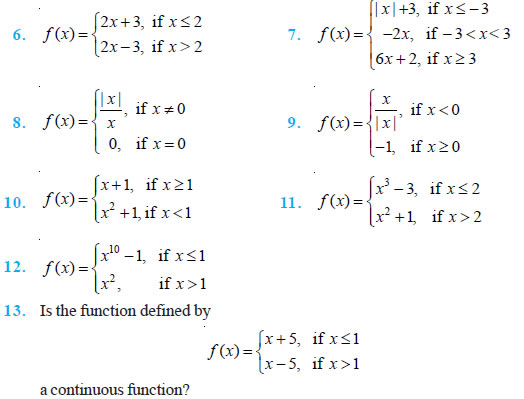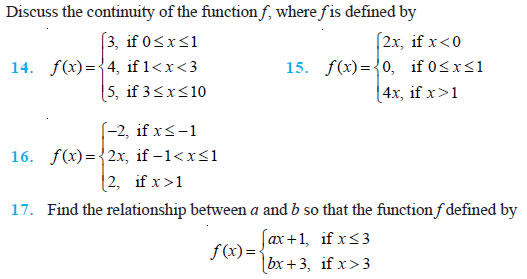Question 18.
For what value of λ is the function defined by ( 2 2 ), if 0 ( ) 4 1, if 0 f x x x x x x ⎧⎪λ − ≤ = + > continuous at x = 0? What about continuity at x = 1?

Question 19. Show that the function defined by g (x) = x – [x] is discontinuous at all integral points. Here [x] denotes the greatest integer less than or equal to x.

Question 20. Is the function defined by f (x) = x2 – sin x + 5 continuous at x = π?

Question 21. Discuss the continuity of the following functions:
(a) f (x) = sin x + cos x
(b) f (x) = sin x – cos x
(c) f (x) = sin x . cos x

Question 22. Discuss the continuity of the cosine, cosecant, secant and cotangent functions.

Question 23. Find all points of discontinuity of f, where sin , if 0 ( ) 1, if 0 x x f x x x x < = + ≥

Question 24. Determine if f defined by 2 sin 1 , if 0 ( ) 0, if 0 x x f x x x ≠ = = is a continuous function?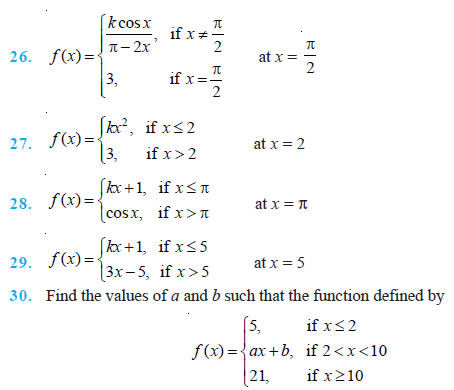is a continuous function.

Question 31.
Show that the function defined by f (x) = cos (x2) is a continuous function.

Question 32. Show that the function defined by f (x) = | cos x | is a continuous function.

Question 33. Examine that sin | x | is a continuous function.

Question 34. Find all the points of discontinuity of f defined by f (x) = | x | – | x + 1 |.

### EXERCISE 5.2

Differentiate the functions with respect to x in Exercises 1 to 8.

Question 1. sin (x2 + 5)

Question 2. cos (sin x)

Question 3. sin (ax + b)

Question 4. sec (tan ( x ))

Question 5. sin ( ) cos ( ) ax b cx d + +

Question 6. cos x3 . sin2 (x5)

Question 7. 2 cot ( x2 )

Question 8. cos( x )

Question 9. Prove that the function f given by f (x) = | x – 1 |, x ∈ R is not differentiable at x = 1.

Question 10. Prove that the greatest integer function defined by f (x) = [x], 0 < x < 3 is not differentiable at x = 1 and x = 2.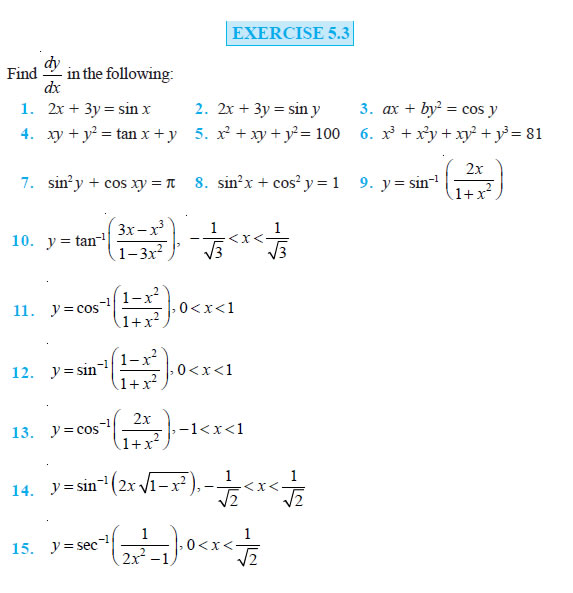### EXERCISE 5.4

Differentiate the following w.r.t. x:

Question 1. sin ex x

Question 2. esin 1 x −

Question 3. ex3

Question 4. sin (tan–1 e–x)

Question 5. log (cos ex)

Question 6. 2 5 ex + ex +... + ex

Question 7. e x , x > 0

Question 8. log (log x), x > 1

Question 9. cos , 0 log x x x >

Question 10. cos (log x + ex), x > 0

### EXERCISE 5.5

Differentiate the functions given in Exercises 1 to 11 w.r.t. x.

Question 1. cos x . cos 2x . cos 3x

Question 2. ( 1)( 2) ( 3)( 4)( 5) x x x x x − − − − −

Question 3. (log x)cos x

Question 4. xx – 2sin x

Question 5. (x + 3)2 . (x + 4)3 . (x + 5)4

Question 6. 1 1 1

Question 7. (log x)x + xlog x

Question 8. (sin x)x + sin–1 x

Question 9. xsin x + (sin x)cos x

Question 10. 2 cos 2 1 1 xx x x x + + −

Question 11. (x cos x)x + 1 (xsin x)x Find dy dx of the functions given in Exercises 12 to 15.

Question 12. xy + yx = 1

Question 13. yx = xy

Question 14. (cos x)y = (cos y)x

Question 15. xy = e(x – y)

Question 16. Find the derivative of the function given by f (x) = (1 + x) (1 + x2) (1 + x4) (1 + x8) and hence find f ′(1).

Question 17. Differentiate (x2 – 5x + 8) (x3 + 7x + 9) in three ways mentioned below:
(i) by using product rule
(ii) by expanding the product to obtain a single polynomial.
(iii) by logarithmic differentiation. Do they all give the same answer?

Question 18. If u, v and w are functions of x, then show that d dx (u. v. w) = du dx v. w + u . dv dx . w + u . v dw dx in two ways - first by repeated application of product rule, second by logarithmic differentiation.

### EXERCISE 5.6

If x and y are connected parametrically by the equations given in Exercises 1 to 10, without eliminating the parameter, Find dy dx .

Question 1.
x = 2at2, y = at4

Question 2. x = a cos θ, y = b cos θ

Question 3. x = sin t, y = cos 2t 4. x = 4t, y = 4 t

Question 5. x = cos θ – cos 2θ, y = sin θ – sin 2θ 6. x = a (θ – sin θ), y = a (1 + cos θ)

Question 7. x = sin3 cos 2 t t , cos3 cos2 y t t =

Question 8. cos log tan 2 x = a = a sin t

Question 9. x = a sec θ, y = b tan θ

Question 10. x = a (cos θ + θ sin θ), y = a (sin θ – θ cos θ)

Question 11. If x asin 1 t , y acos 1 t , show that dy y dx x

### EXERCISE 5.7

Find the second order derivatives of the functions given in Exercises 1 to 10.

Question1.
x2 + 3x +

Question 2. x20

Question 3. x . cos x

Question 4. log x

Question 5. x3 log x

Question 6. ex sin 5x

Question 7. e6x cos 3x

Question 8. tan–1 x

Question 9. log (log x)

Question 10. sin (log x)

Question 11. If y = 5 cos x – 3 sin x, prove that 2 2 d y y 0 dx + =

Question 12. If y = cos–1 x, Find 2 2 d y dx in terms of y alone.

Question 13. If y = 3 cos (log x) + 4 sin (log x), show that x2 y2 + xy1 + y = 0

Question 14. If y = Aemx + Benx, show that 2 2 d y (m n) dy mny 0 dx dx − + + =

Question 15. If y = 500e7x + 600e– 7x, show that 2 2 d y 49y dx =

Question 16. If ey (x + 1) = 1, show that 2 2 2 d y dy dx dx =

Question 17. If y = (tan–1 x)2, show that (x2 + 1)2 y2 + 2x (x2 + 1) y1 = 2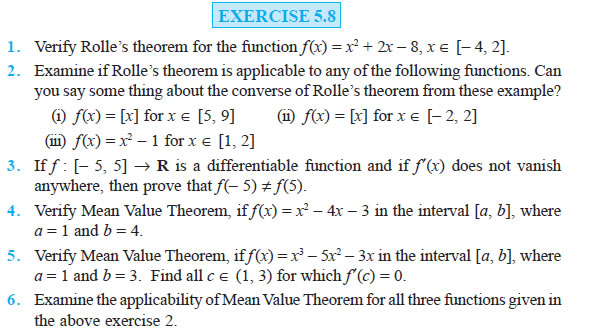### NCERT Books Free Pdf Download for Class 5, 6, 7, 8, 9, 10 , 11, 12 Hindi and English Medium

 Mathematics Biology Psychology Chemistry English Economics Sociology Hindi Business Studies Geography Science Political Science Statistics Physics Accountancy